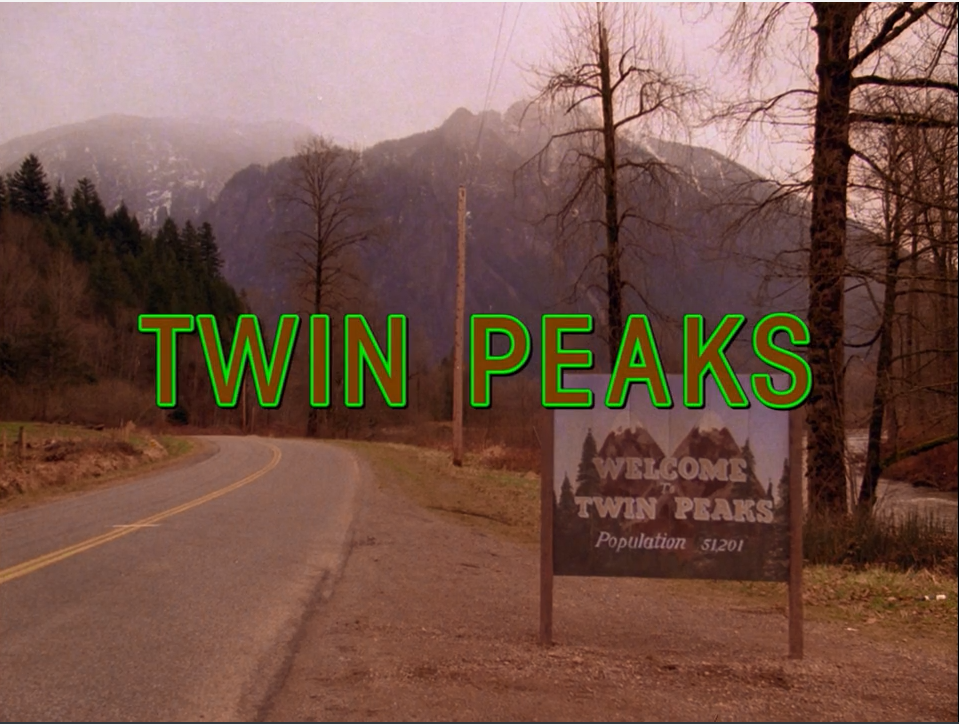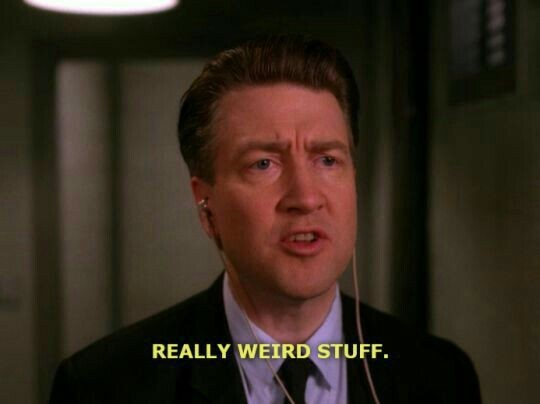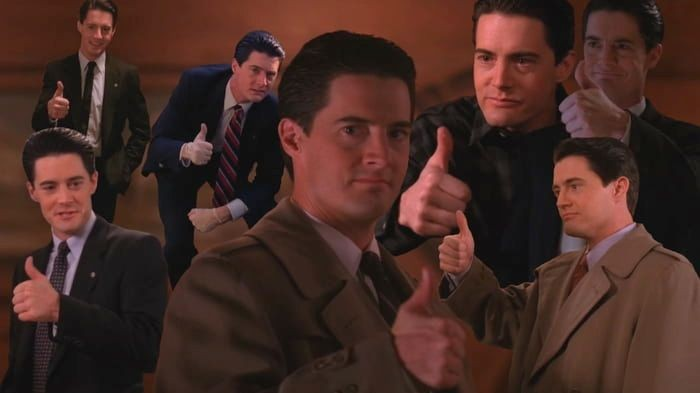# 迷人的 Twin Peaks，迷人的 大卫林奇self.__wrap_n=self.__wrap_n||(self.CSS&&CSS.supports("text-wrap","balance")?1:2);self.__wrap_b=(e,n,t)=>{t=t||document.querySelector(`[data-br="\${e}"]`);let r=t.parentElement,o=f=>t.style.maxWidth=f+"px";t.style.maxWidth="";let c=r.clientWidth,a=r.clientHeight,s=c/2-.25,i=c+.5,p;if(c){for(o(s),s=Math.max(t.scrollWidth,s);s+1<i;)p=Math.round((s+i)/2),o(p),r.clientHeight===a?i=p:s=p;o(i*n+c*(1-n))}t.__wrap_o||(typeof ResizeObserver!="undefined"?(t.__wrap_o=new ResizeObserver(()=>{self.__wrap_b(0,+t.dataset.brr,t)})).observe(r): false&&0)};self.__wrap_n!=1&&self.__wrap_b(":Rarbb9mba:",1)"双峰镇，人人都在外遇，人人都是名侦探，人人都想插一脚，人人都以为自己在恋爱，大部分都是变态。"• 锈湖里的 Mr. Owl、侦探 Dale 和各种特效、音效、美术风格

• 奇异人生里的 FireWalk 乐队（对应双峰里的 Fire Walk With Me

• Chloe 的车牌 TWN PKS

• Chloe 妈妈开的 Two Whales Diner（对应 Double R Diner ）

• 都是用小镇交际花失踪/遇难作为 麦格芬 引起的一系列事情​ 第二季就感觉拉垮了，有点拖沓了，也是后面才知道是幕后制作的原因，林奇的理念以及预算问题导致剧被 ABC 砍了（BTW，第二季的 Annie 真的可惜了）。电影 Fire Walk With Me 和第三季就真的满满的 林奇感 了。电影版给我的惊喜就是 David Bowie 的出演，他在里面饰演的是个重要人物 —— Agent Phillip Jeffries，很可惜第三季没有他了。